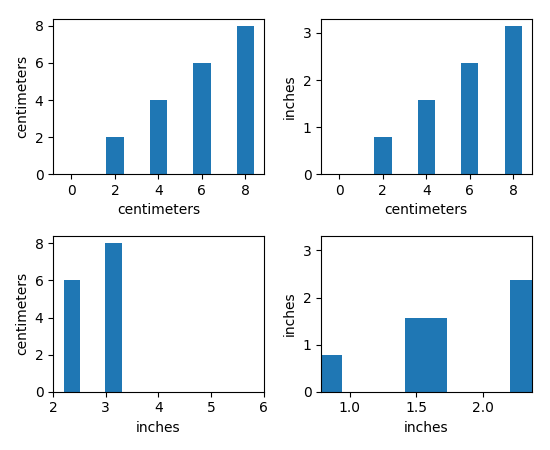You are reading an old version of the documentation (v2.0.2). For the latest version see https://matplotlib.org/stable/Travis-CI:# units example code: bar_demo2.py¶```"""
===================
Bar demo with units
===================

A plot using a variety of centimetre and inch conversions. This example shows
how default unit introspection works (ax1), how various keywords can be used to
set the x and y units to override the defaults (ax2, ax3, ax4) and how one can
set the xlimits using scalars (ax3, current units assumed) or units
(conversions applied to get the numbers to current units).

"""
import numpy as np
from basic_units import cm, inch
import matplotlib.pyplot as plt

cms = cm * np.arange(0, 10, 2)
bottom = 0 * cm
width = 0.8 * cm

fig, axs = plt.subplots(2, 2)

axs[0, 0].bar(cms, cms, bottom=bottom)

axs[0, 1].bar(cms, cms, bottom=bottom, width=width, xunits=cm, yunits=inch)

axs[1, 0].bar(cms, cms, bottom=bottom, width=width, xunits=inch, yunits=cm)
axs[1, 0].set_xlim(2, 6)  # scalars are interpreted in current units

axs[1, 1].bar(cms, cms, bottom=bottom, width=width, xunits=inch, yunits=inch)
axs[1, 1].set_xlim(2 * cm, 6 * cm)  # cm are converted to inches

fig.tight_layout()
plt.show()
```

Keywords: python, matplotlib, pylab, example, codex (see Search examples)